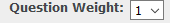KnowledgebaseSet Weights for Questions Posted by , Last modified by Prasad De Silva on 07 October 2020 02:45 PM This will allow admin to specify weights for the questions at the time of creating. Weights are ranging from 1 to 5 and weight 5 carriers the maximum weight. When the exam is graded the score will depend on the weight of questions. Setup From the Admin site - Go to Curriculum – Course – Lesson – Objective When you create your question, you can select the weight for that question. Default value set to 1.Logic When the exam is graded the score will depend on the weight of questions example Assume Q1 - weight = 2 Q2 - weight = 3 Q3 - weight = 4 Q4 - weight = 1 Q5 - weight = 5 Let's assume student got these 5 questions in a exams got Q1, Q2, Q3 correct and Q4 and Q5 wrong So the score should be (sum of weights of all question the student got right) * 100 / (sum of weights of all question the student got in exam) Ex (2+3+4)X100/(2+3+4+1+5) = 9 X 100 / 15 = 60%(4 vote(s)) Helpful Not helpful